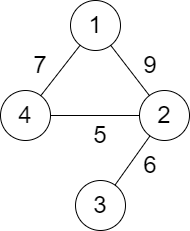# Solved! Leetcode 2492. Minimum Score of a Path Between Two Cities

### Minimum Score of a Path Between Two Cities

You are given a positive integer n representing n cities numbered from 1 to n. You are also given a 2D array roads where roads[i] = [ai, bi, distancei] indicates that there is a bidirectional road between cities ai and bi with a distance equal to distancei. The cities graph is not necessarily connected.

The score of a path between two cities is defined as the minimum distance of a road in this path.

Return the minimum score of a path between cities 1 and n.

Note:

• A path is a sequence of roads between two cities.
• It is allowed for a path to contain the same road multiple times, and you can visit cities 1 and n multiple times along the path.
• The test cases are generated such that there is at least one path between 1 and n.

Example 1:Input: n = 4, roads = [[1,2,9],[2,3,6],[2,4,5],[1,4,7]]
Output: 5
Explanation: The path from city 1 to 4 with the minimum score is: 1 -> 2 -> 4. The score of this path is min(9,5) = 5.
It can be shown that no other path has less score.

Example 2:Input: n = 4, roads = [[1,2,2],[1,3,4],[3,4,7]]
Output: 2
Explanation: The path from city 1 to 4 with the minimum score is: 1 -> 2 -> 1 -> 3 -> 4. The score of this path is min(2,2,4,7) = 2.

Constraints:

• 2 <= n <= 105
• 1 <= roads.length <= 105
• 1 <= ai, bi <= n
• ai != bi
• 1 <= distancei <= 104
• There are no repeated edges.
• There is at least one path between 1 and n.